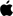# Macc++ question

#### chris200x9

##### macrumors 6502a
Original poster
how would I go about creating a program to calculate shipping charges?

i.e. If the order weight is less than 8 ounces, there is a ﬂat rate charge of \$2.00.
If the order weight is between 8 ounces and 32 ounces, the charge is \$2.00
plus \$0.75 for each ounce, or part thereof, over 8 ounces.
If the order weight is greater than 32 ounces, the charge is \$20.00 plus
\$1.15 for each ounce over 32 ounces.

I would now how to the only problem is it excepts pounds and ounces

so someone can put in 0.5 pounds and it should be 2.00 also

#### hankolerd

##### macrumors 6502
how would I go about creating a program to calculate shipping charges?

i.e. If the order weight is less than 8 ounces, there is a ﬂat rate charge of \$2.00.
If the order weight is between 8 ounces and 32 ounces, the charge is \$2.00
plus \$0.75 for each ounce, or part thereof, over 8 ounces.
If the order weight is greater than 32 ounces, the charge is \$20.00 plus
\$1.15 for each ounce over 32 ounces.

I would now how to the only problem is it excepts pounds and ounces

so someone can put in 0.5 pounds and it should be 2.00 also

1) make sure whatever the value is it is in ounces
2)
---if (weight > 8)
---{
---------if (weight > 32)
---------{
--------------price = 20.00
--------------weight -= 32
--------------while (weigh != 0)
--------------{
------------------price += 1.15
------------------weight -= 1
---------------}
---------}
---------else
---------{
-------------price = 2.00
-------------weight -= 8
-------------while (weight != 0)
-------------{
------------------price += .75
------------------weight -= 1
-------------}
--------}
--}
--else
------price = 2.00

hope this design helpsEDIT: WHY DOESNT THE SPACES EVER SHOW UP!!!

Comment

#### stupidregister

##### macrumors member
hankolerd, you could use the code tags to preserve formatting.

Code:
``````if (weight > 8)
{
if (weight > 32)
{
price = 20.00
weight -= 32
while (weigh != 0)
{
price += 1.15
weight -= 1
}
}
else
{
price = 2.00
weight -= 8
while (weight != 0)
{
price += .75
weight -= 1
}
}
}
else
price = 2.00``````

Comment

#### Aranince

##### macrumors 65816
hankolerd, you could use the code tags to preserve formatting.

Code:
``````if (weight > 8)
{
if (weight > 32)
{
price = 20.00
weight -= 32
while (weigh != 0)
{
price += 1.15
weight -= 1
}
}
else
{
price = 2.00
weight -= 8
while (weight != 0)
{
price += .75
weight -= 1
}
}
}
else
price = 2.00``````
That should work...just don't forget the semicolons!

Comment

##### macrumors newbie
Code:
``````float shippingCharge(float pounds, float ounces)
{
float weight = pounds * 16 + ounces;

// weight from 0 to 8 ounces
if (weight <= 8.0)
return 2.0;

// weight from 8 to 32 ounces
if (weight <= 32.0)
return 2.0 + 0.75 * (weight - 8.0);

// weight over 32 ounces
return 20.0 + 1.15 * (weight - 32.0);
}``````

Comment

#### toddburch

##### macrumors 6502a
Based on this requirement:

plus \$0.75 for each ounce, or part thereof,
Shouldn't this be considered:

Code:
``````float shippingCharge(float pounds, float ounces)
{
float weight = pounds * 16 + ounces;
[color=red]if ((int) weight < weight) { weight = (int) (weight+1) } ; [/color]
...
}``````
(not syntax checked)

Todd

Comment

##### macrumors newbie
Good catch. I'd prefer
Code:
``float weight = ceil(pounds * 16.0 + ounces);``
though.

Comment

#### toddburch

##### macrumors 6502a
Good catch. I'd prefer
Code:
``float weight = ceil(pounds * 16.0 + ounces);``
though.
Yep. that's better.

Comment

• PinkyMacGodess
2
Replies
2
Views
431
• tk_mac
1
Replies
1
Views
488
Register on MacRumors! This sidebar will go away, and you'll see fewer ads.